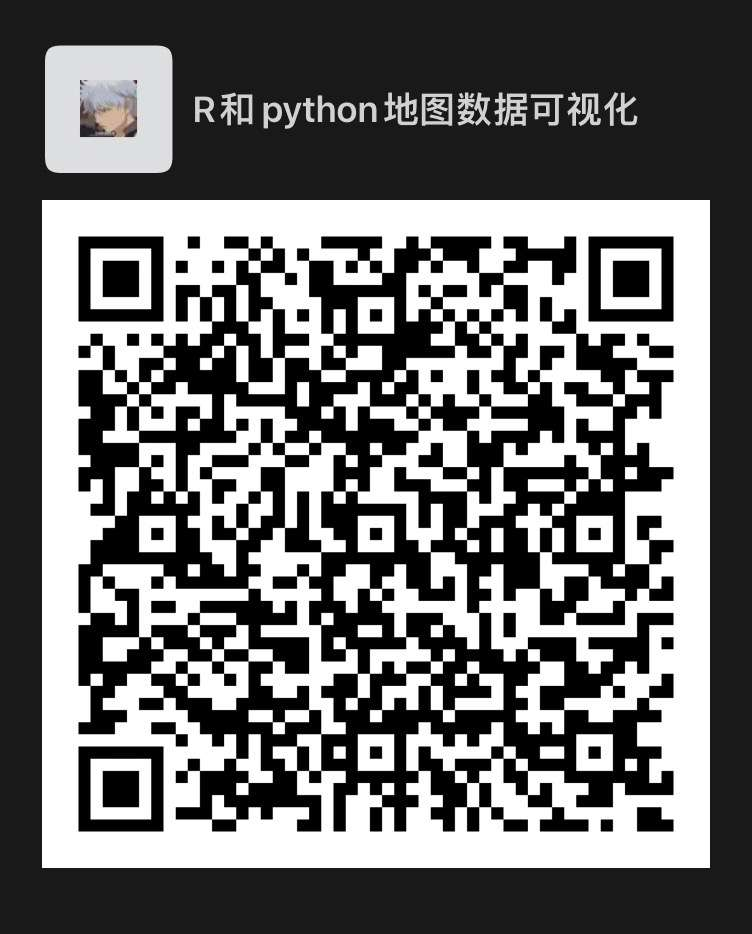墨滴yuanzhoulvpi

2021/10/30  阅读：57  主题：橙心

# 中国地图数据分享

## 地理数据内容介绍

1. 全国地图；该有的都有，懂得都懂。
2. 市、区（县）。

## 使用方法

### PYTHON版本使用

``import numpy as npimport pandas as pdimport geopandas as gpd import matplotlib.pyplot as plt map_data = gpd.read_file("中国地图数据文件夹/100000_中华人民共和国_full.json")map_data['coords'] = map_data['geometry'].apply(lambda x: x.representative_point().coords[:])plt.rcParams['font.sans-serif'] = ['Arial Unicode MS']fig, ax = plt.subplots(figsize=(10,7), dpi=300)map_data.plot(ax=ax, column='name')for idx, row in map_data.iterrows():    plt.annotate(text=row['name'], xy=row['coords'],                 horizontalalignment='center',size=4)plt.show()``

### R版本使用

``library(tidyverse)library(sf)#如果电脑显示字体没问题，那就不需要这块代码library(showtext)showtext_auto()file_name <- "中国地图数据文件夹/100000_中华人民共和国_full.json"map_data <- sf::read_sf(file_name)map_data %>% mutate(id = rnorm(n = n())) %>%  ggplot(aes(geometry = geometry, fill = id)) +  geom_sf(show.legend = FALSE) +  geom_sf_text(aes(label = name), size = 2) + theme_bw() +  labs(fill = "", x = '', y = '') +  theme(panel.border = element_blank()) +  scale_fill_viridis_c()``

## 如何获得这个数据

1. 转发本推文到朋友圈，点赞超过20个。然后将朋友圈截图发送到公众号。

2. 可以选择途径：一个是可以提供百度网盘链接。一个是邮件分享发送（需要填写邮件地址）。数据会有延迟，但是不会迟到～

## 更多

1. 微信群：2. 微信群过期的话，可以添加我的微信：yuanzhoulvpi

2021/10/30  阅读：57  主题：橙心